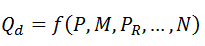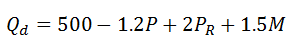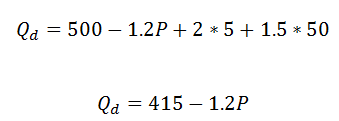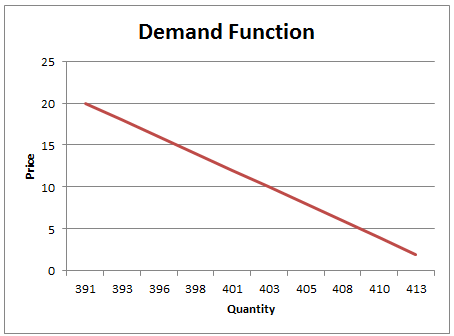# Demand Function and Demand Curve

The two fundamental principles in microeconomics are the principles of demand and supply of goods and services. At any point of time, the various goods and services will have a certain demand and supply that will depend on a variety of factors.

### Demand

Demand refers to the ability and the willingness of consumers to buy certain quantities of goods and services at a given price during a given time period. Demand of a product is affected by many factors such as the cost of production, its price compared to other alternative products, or the income levels of consumers. All these are called the determinants of demands.

For any product, we can calculate the quantity demanded as a function of various factors influencing the demand. The quantity demanded (Qd) is the amount of a good or service consumers are willing & able to purchase during a given period of time.

Factors Affecting Quantity Demanded

The common factors affecting the quantity demanded are:

• Price of good or service (P)
• Incomes of consumers (M)
• Prices of related goods & services (PR)
• Expected future price of product (Pe)
• Number of consumers in market (N)
• Taste patterns of consumers (Á)

### General Demand Equation

The general demand function with the above determinants will be as follows:A simple demand function for product X is presented below:Here 500 is a cumulative of all the factors that are not specified above. The quantity demanded is inversely related to price of the products, i.e., if prices fall, the demand will increase. The quantity demanded is positively related to the price of related goods, i.e., if the price of related goods increases, the quantity demanded for product X will increase. The quantity demanded is also positively related to the income of consumers, i.e., if the income is more, the quantity demanded will be more.

When the quantity demanded is expressed only as a function of the price of the product, it is called a demand function.

Let’s say the price of substitute products is 5 and the income is 50, the above equation can be rewritten as follows:Other factors remaining constant, the quantity demanded will be 415 - 1.2P.

Note that quantity is a linear function of price and the quantity is inversely proportional to price. For every \$1 increase in price of the product, the quantity demanded will reduce by 1.2 units.

### Demand Curve

The information from the demand function can be plotted as a simple graph with quantity demanded on x-axis and price on y-axis. This is called a demand curve.

The equation plotted is the inverse demand function, P = f(Qd)

A point on the demand curve can be interpreted as follows:

• Maximum amount of a good that will be purchased for a given price
• Maximum price consumers will pay for a specific amount of the goodThe demand curve illustrates the law of demand. The law of demand states that when the price of a good rises, and everything else remains the same, the quantity of the good demanded will fall.